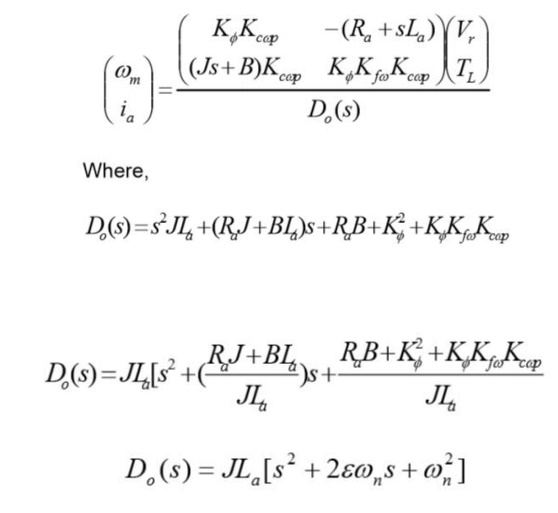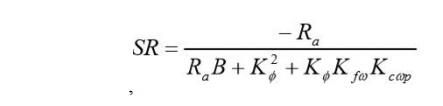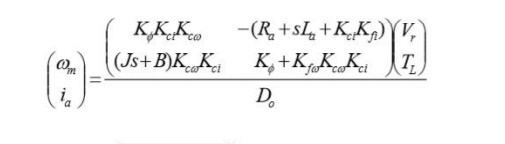Home | | Solid State Drives | Closed Loop Control with Current and Speed Feedback

# Closed Loop Control with Current and Speed Feedback

Closed loop control improves on the drives performance by increasing speed of response and improving on speed regulation.

Closed Loop Control With Current And Speed Feedback

Closed loop control improves on the drives performance by increasing speed of response and improving on speed regulation. So the functions of closed loop control is that ωn is increased, ε is reduced, ts is reduced, and Speed Regulation (SR) is reduced. A closed loop speed control scheme is shown belowSchematic Diagram of the Closed Loop Speed Control

Where, Kfω is the tachometer feedback gain

Kc(s) is the speed controller gain

Kr(s) is the armature voltage regulator gain

The block diagram representation of the control configuration is shown below.Block Diagram of the Closed Loop Speed Control.If the tachometer loop does not contain a filter, the feedback gain can be a constant designated as Kfw

Kcw(s) = Kcwp + KcwI/ s +sKcwd

Where,

Kcwd is the proportional gain component of Kcw(s)

Kcwp is the integral gain component of Kcw(s) & Kcwd

Three possible controller configurations are possible:

1.For KcwI & Kcwd zero Kcw(S) = Kcwp Which is a Proportional Controller

2. For Kcwp & Kcwd zero Kcw(S) = KcwI/S Which is an Integral Controller

3. For Kcwd zero Kcw(S) = Kcwp+ KcwI/S Which is a Proportional Integral Controller

Now taking the Proportional Controller as a case study, with Kcw(S) = Kcwp , the dynamic equation is,Last Equation is a second order system

The Natural Frequency of Oscillation, ωn  is,This is always higher than the open loop case due to the factor Kф ,K, KcѠp

The Damping Ratio, ε , isThis is lower than in the open loop case due to the increase in ωn

Speed Regulation (SR) is also derived asSR is also lower than in the open loop case due to the factor Kф ,K, KcѠp . This is an indication of a better drive performance.

## Inner Current Loop Control

Improvement in speed control can be obtained with Inner Current Loop Control method, whereby armature current is fed back to the input. A closed loop speed control scheme with inner current control is shown in Figure.

Designating K1ci+Kr=Kci the block diagram representation of the control configuration is shown in Figure.

The dynamic equation is,Study Material, Lecturing Notes, Assignment, Reference, Wiki description explanation, brief detail
Solid State Drives : Design of Controllers For Drives : Closed Loop Control with Current and Speed Feedback |